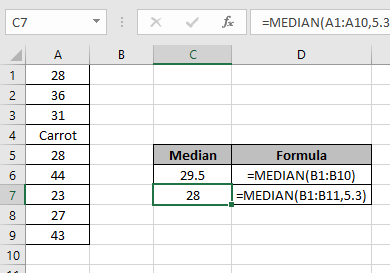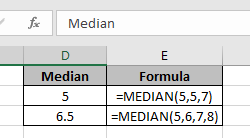# How to use the MEDIAN function in ExcelIn this article, we will learn How to use the MEDIAN function in Excel.

What is the median of values ?

Median of the values mean, if the values are sorted in ascending or descending order and then picked out the middle number from the values. That is the median. For example in a list of 5 numbers 1, 5, 10, 50 & 75. The middle number position is calculated using a simple formula i.e. total numbers of values + divided by 2. Here 5/2 comes out to be 2.5, position of a value cannot be in decimal, so we consider 3rd position as the median value which is 10.

You must be wondering what happens if the total number of values are even. Then there are two middle numbers. What to choose then. Just take the average of those numbers. That will be the median value. For example if given a list of 6 numbers 1, 5, 10, 50, 75 & 200. Here 10 and 50 both are middle numbers in a list of values. So the average of 10 & 50 is (10+50)/2 which is 30. So 30 is the median value. Let's understand how MEDIAN function syntax works and an example to illustrate the function usage in Excel.

MEDIAN Function in Excel

The MEDIAN function in Excel returns the median value from the given list of numbers. The function know what to do in case of count of odd numbers and in count of even numbers.

MEDIAN Function syntax:

 =MEDIAN(array_reference)

array_reference : List of values given as array like values in range C5 to C10. Given as C5:C10

Example :

All of these might be confusing to understand. Let's understand how to use the function using an example. Here first we test the function for smaller results.

Median of values : 5, 5, 7 : 5 (middle position number)

Median of values : 5, 6, 7, 8 : (6+7)/2 = 6.5 (average value of 2 middle numbers 6 and 7)Now we take a list of values including text values. And see how MEDIAN function ignores text values in a list of numbers.

Now use the excel Median function on the range or array of valuesIn the C7 cell, we just added one more number (5.3) in the range of numbers (A1:A10).

Here we got the median of the range of values(A1:A10).

As you can see from the above examples, how to use median function in Excel.

Here are all the observational notes using the MEDIAN function in Excel
Notes :

1. The function only works with numbers.
2. The function ignores only text values. Be careful using along with other number format values like date, time, percentage values.
3. The function return # NUM! Error if no value is given.
4. The function returns the value itself if there is only one numerical value.

Hope this article about How to use the MEDIAN function in Excel is explanatory. Find more articles on calculating values and related Excel formulas here. If you liked our blogs, share it with your friends on Facebook. And also you can follow us on Twitter and Facebook. We would love to hear from you, do let us know how we can improve, complement or innovate our work and make it better for you. Write to us at info@exceltip.com.

Related Articles :

How to use the ROUND function in Excel : rounds off the given number to the nearest num_digit decimal using the ROUND function in Excel

How to use the MOD function in Excel : returns the Most repeated value in a list of numbers called MOD value. MOD function explained with an example here.

How to use the MEAN or AVERAGE function in Excel : returns the average of the list of numbers. Mean of numbers and average of numbers is the same in Excel. Use the MEAN function to get the average of values in Excel.

How to use Excel F.TEST Function in Excel : The F.TEST Function is used to calculate F statistic of two samples in excel internally and returns the two tailed probability of the F statistic under Null Hypothesis.

How To Use Excel T.TEST Function in Excel : The T.TEST function is used to determine the confidence of an analysis in Excel. Mathematically, it is used to know if the mean of the two samples are equal or not. T.TEST function is used to accept or reject the null hypothesis.

How to use the DEVSQ Function in Excel : DEVSQ function is a built-in statistical function to calculate the sum of squared deviations from the mean or average of the given distribution provided.

How to calculate Standard Deviation in Excel : To calculate the standard deviation we have different functions in Excel. The standard deviation is the square root of the variance value but It tells more about the dataset than variance.

How to use the VAR function in Excel : Calculate the variance for the sample dataset in excel using the VAR function in Excel.

Popular Articles :

How to use the IF Function in Excel : The IF statement in Excel checks the condition and returns a specific value if the condition is TRUE or returns another specific value if FALSE.

How to use the VLOOKUP Function in Excel : This is one of the most used and popular functions of excel that is used to lookup value from different ranges and sheets.

How to use the SUMIF Function in Excel : This is another dashboard essential function. This helps you sum up values on specific conditions.

How to use the COUNTIF Function in Excel : Count values with conditions using this amazing function. You don't need to filter your data to count specific values. Countif function is essential to prepare your dashboard.

Terms and Conditions of use

The applications/code on this site are distributed as is and without warranties or liability. In no event shall the owner of the copyrights, or the authors of the applications/code be liable for any loss of profit, any problems or any damage resulting from the use or evaluation of the applications/code.